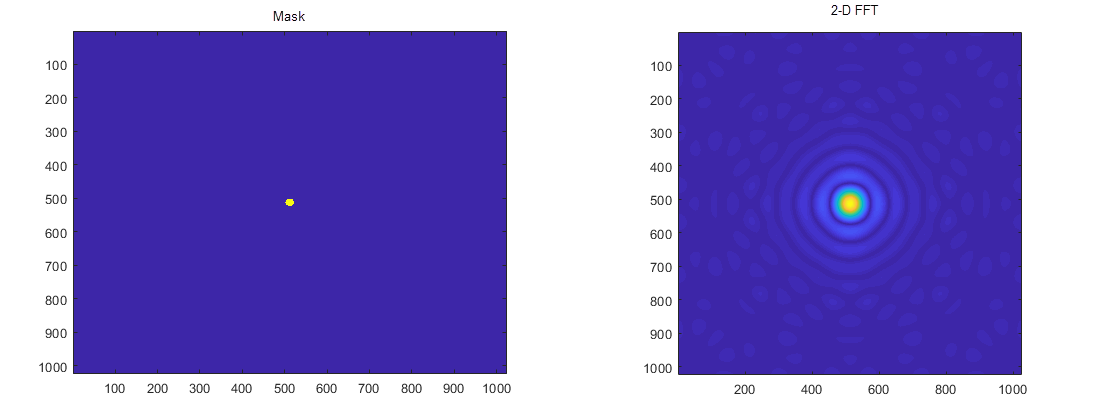## FFT Example

This example shows how a two-dimensional Fourier transform can be used on an optical mask to compute its diffraction pattern. Create a logical array that defines an optical mask with a small, circular aperture.

```n = 2^10; % size of mask M = zeros(n); I = 1:n; x = I-n/2; % mask x-coordinates y = n/2-I; % mask y-coordinates [X,Y] = meshgrid(x,y); % create 2-D mask grid R = 10; % aperture radius A = (X.^2 + Y.^2 <= R^2); % circular aperture of radius R M(A) = 1; % set mask elements inside aperture to 1 figure imagesc(M) % plot mask axis image DP = fftshift(fft2(M)); imagesc(abs(DP)) axis image```### Prepare `myFFT` for Kernel Creation

Create an entry-point function `myFFT` that computes the 2-D Fourier transform of the mask by using the `fft2` function. Use the `fftshift` function to rearrange the output so that the zero-frequency component is at the center. To map this function to a GPU kernel, place the `coder.gpu.kernelfun` pragma within the function.

```function [DP] = myFFT(M) coder.gpu.kernelfun(); DP = fftshift(fft2(M));```

### Generated CUDA Code

When you generate CUDA® code, GPU Coder™ creates function calls (`cufftEnsureInitialization`) to initialize the cuFFT library, perform FFT operations, and release hardware resources that the cuFFT library uses. A snippet of the generated CUDA code is:

```void myFFT(myFFTStackData *SD, const real_T M, creal_T DP) { ... cudaMemcpy((void *)gpu_M, (void *)M, 8388608ULL, cudaMemcpyHostToDevice); myFFT_kernel1<<<dim3(2048U, 1U, 1U), dim3(512U, 1U, 1U)>>>(gpu_M, gpu_b); cufftEnsureInitialization(1024, CUFFT_D2Z, 1024, 1024); cufftExecD2Z(*cufftGlobalHandlePtr, (cufftDoubleReal *)&gpu_b, (cufftDoubleComplex *)&gpu_y1); ... myFFT_kernel2<<<dim3(2048U, 1U, 1U), dim3(512U, 1U, 1U)>>>(gpu_y1, gpu_y); cufftEnsureInitialization(1024, CUFFT_Z2Z, 1024, 1024); cufftExecZ2Z(*cufftGlobalHandlePtr, (cufftDoubleComplex *)&gpu_y, (cufftDoubleComplex *)&gpu_DP, CUFFT_FORWARD); ... cufftEnsureDestruction(); ... }```

The first `cudaMemcpy` function call transfers the 1024x1024 double-valued input `M` to the GPU memory. The `myFFT_kernel1` kernel performs pre-processing of the input data before the cuFFT library calls. The two-dimensional Fourier transform call `fft2` is equivalent to computing `fft(fft(M).').'`. Because batched transforms generally have higher performance compared to single transforms, GPU Coder has two 1-D cuFFT calls `cufftExecD2Z` to compute the double-precision real-to-complex forward transform of the input `M` followed by `cufftExecZ2Z` to perform the double-precision complex-to-complex transform of the result. The `cufftEnsureDestruction()` call destroys and frees all GPU resources associated with the cuFFT library call.